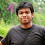## Monday, July 8, 2013

### SIGINT CTF - crypto 200 - RSA - [Team xbios]

We were given two files for this challenge - python script used to generate RSA keypair and authorized_keys. Authorized_keys is the public key which has the n and e value. Below is the code
```#!/usr/bin/env python

from time import time
from os import system
from Crypto.PublicKey import RSA

SEED = int(time())

def randfunc(n):
def rand():
global SEED
ret = SEED*0x1333370023004200babe004141414100e9a1192355de965ab8cc1239cf015a4e35 + 1
SEED = ret
return (ret >> 0x10) & 0x7fff
ret = ""
while len(ret) < n:
ret += chr(rand() & 0xff)
return ret

keypair = RSA.generate(1024, randfunc)

with open("pub", "w") as pubfile, open("id_rsa", "w") as privfile:
privfile.write(keypair.exportKey())
pubfile.write(keypair.publickey().exportKey())

system("ssh-keygen -m PKCS8 -i -f pub > id_rsa.pub && rm pub")
```
Looking at the code we can see that, current time is used as SEED value during key generation. To solve the challenge

[*] Find the SEED value used in key generation
[*] Generate the private key pair for the given public key
[*] Copy the generated private key to ~/.ssh/id_rsa. Now we can prove our identity to the remote machine and perform a login

Bruteforcing the SEED value was a bit of pain. After some failures, finally we decided to bruteforce the SEED value by going behind the start of CTF time ie
```SEED = 1373040000
GMT: Fri, 05 Jul 2013 16:00:00 GMT
```
The n value was extracted from authorized key as
```
```
Below is the script we used for bruteforce
```#!/usr/bin/env python

from Crypto.PublicKey import RSA
from sys import exit

SEED = 1373040000
counter = 0

def randfunc(n):
global SEED_Brute
ret = ""

while len(ret) < n:
tmp = SEED_Brute*0x1333370023004200babe004141414100e9a1192355de965ab8cc1239cf015a4e35+1
SEED_Brute = tmp
tmp = (tmp >> 0x10) & 0x7fff
ret += chr(tmp & 0xff)
return ret

while 1:
SEED_Brute = SEED - counter
keypair = RSA.generate(1024, randfunc)
if keypair.n == target:
print keypair.p
print keypair.q
print (SEED - counter)
exit(0)
counter += 1
```
After sometime we got the following values:
```p = 10196183246368760603869192593971202143897281417220455881063414616103901438182656326076501376638806928762094749150020638960102206987607293047096627515275223
q = 13097286606179453667665592444299109782484218865253457545521978739889248320232481682880143106432871469494586765663594908396375009598486558938138835723794021
SEED = 1373038672
```
Now generate the private key and place it in ~/.ssh/id_rsa and connect to the challenge machine
```challenge@ubuntu:~\$ ls
flag.txt
challenge@ubuntu:~\$ cat flag.txt
SIGINT_some_people_pay_100_euro_for_this
```
Flag for the challenge is SIGINT_some_people_pay_100_euro_for_this

Update:

Well, I tried out the optimization as mentioned in the comment section. Thought of making a small note on it.

[*] rand() function is linear congruential generator of form (((SEED * 0x1333370023004200babe004141414100e9a1192355de965ab8cc1239cf015a4e35) + 1 ) >> 16 ) mod 2^15
[*] After computing ((SEED * 0x1333370023004200babe004141414100e9a1192355de965ab8cc1239cf015a4e35) + 1 ), the output is shifted by 16 bits then mod 2^15 is done. So its enough to consider only 32 bits from left, since bits 31 to 16 is brought to bit position 15 to 0
[*] Multiplication is nothing but repeated addition. Even if you add 0x1333370023004200babe004141414100e9a1192355de965ab8cc1239cf015a4e35 'n' number of times, only final 32 bits matter to us. So we can do
```>>> hex(0x1333370023004200babe004141414100e9a1192355de965ab8cc1239cf015a4e35 & 0xffffffff)
'0x15a4e35L'
Thats exactly 25 bits
```
[*] If SEED is greater than 2**32, the value of multiplication is going to cycle back. So its enough to consider only the 4 bytes of SEED
```>>> hex(0x15a4e35 * 1)
'0x15a4e35'
>>> hex(0x15a4e35 * 2)
'0x2b49c6a'
>>> hex(0x15a4e35 * (2**32+1))
'0x15a4e35015a4e35'
>>> hex(0x15a4e35 * (2**32+2))
'0x15a4e3502b49c6a'
>>> hex(0x15a4e35 * (2**32+1) & 0xffffffff)
'0x15a4e35'
>>> hex(0x15a4e35 * (2**32+2) & 0xffffffff)
'0x2b49c6a'
```
[*] Thus we can reduce the multiplier to 0x15a4e35 and SEED to 4 bytes in LCG. Since only 8 bits of LCG is used, further optimization can be achieved by using only 3 bytes of multiplier and SEED

1.1.